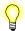# logb(), logbf()

## Synopsis:

```#include <math.h>

double logb ( double x );

float logbf ( float x );```

## Arguments:

x
The number that you want to compute the radix-independent exponent of.

## Library:

libm

Use the -l m option to qcc to link against this library.

## Description:

The logb() and logbf() functions compute the exponent part of x, which is the integral part of:

logr |x|

as a signed floating point value, for nonzero finite x, where r is the radix of the machine's floating point arithmetic.

## Returns:

The binary exponent of x, a signed integer converted to double-precision floating-point.

If x is: logb() returns:
0.0 -HUGE_VAL (errno is set to EDOM)
<0.0 -HUGE_VAL (errno may be set to ERANGE)
±infinity +infinityIf an error occurs, these functions return 0, but this is also a valid mathematical result. If you want to check for errors, set errno to 0, call the function, and then check errno again. These functions don't change errno if no errors occurred.

## Examples:

```#include <stdio.h>
#include <errno.h>
#include <inttypes.h>
#include <math.h>
#include <fpstatus.h>

int main(int argc, char** argv)
{
double a, b;

a = 0.5;
b = logb(a);
printf("logb(%f) = %f (%f = 2^%f) \n", a, b, a, b);

return(0);
}```

produces the output:

`logb(0.500000) = -1.000000 (0.500000 = 2^-1.000000)`

## Classification:

Safety:
Cancellation point No
Interrupt handler No
Signal handler No